# Google CTF 2018 (Quals) – writeup JS safe 2.0

The qualifications for the Google Capture The Flag 2018 (ctftime.org) ran from 23/06/2018, 00:00 UTC to 24/06/2018 23:59 UTC.The CTF was worked out very well. There have been plenty of interesting and creative challenges.

This time I decided to focus on the category web and managed to solve the challenge JS safe 2.0, which was the easiest one of the web-challenges based on the amount of solves. Nevertheless it really took my some time to dodge all the pitfalls I stumbled upon while solving the challenge.

# JS safe 2.0 (121 pts – 103 solves)

The writeup is divided into the following sections:

### 1. Challenge description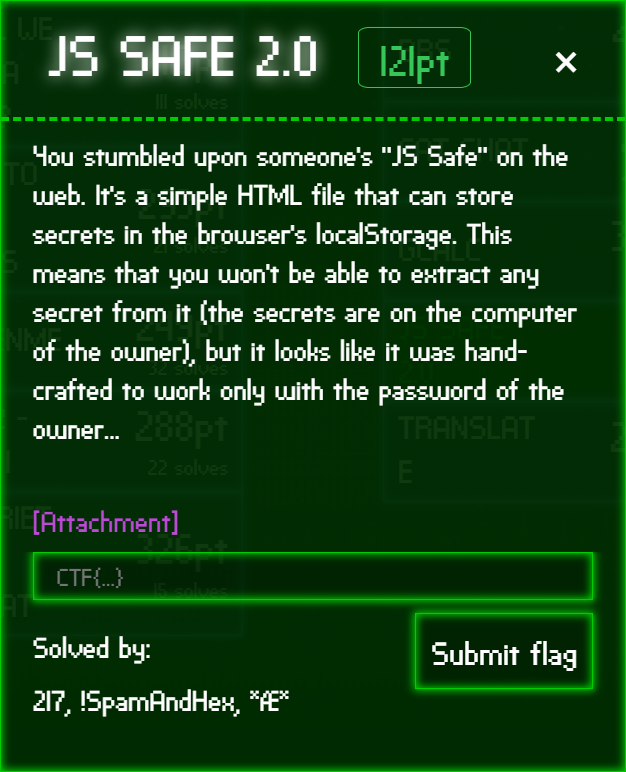The challenge is an offline client-side challenge. The attached zip-file contains a single html-file called `js_safe_2.html`. The html-page displays an input field, in which a password should be entered:When entering an incorrect password the message `Access Denied` is displayed at the bottom of the page: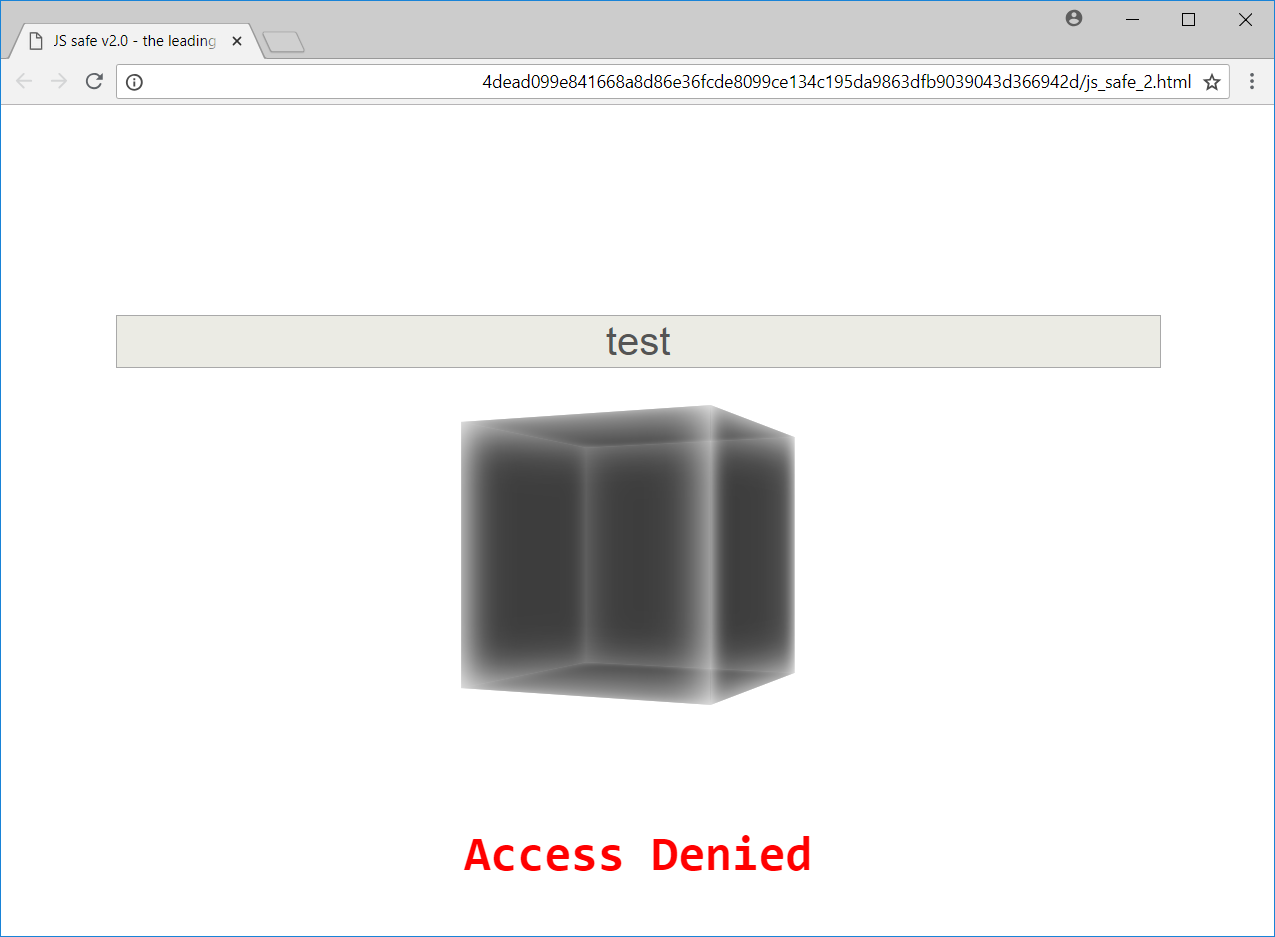### 2. Source Code

Let’s have a look at the source-code:

```<!DOCTYPE html>
<html>
<meta charset="utf-8">
<title>JS safe v2.0 - the leading localStorage based safe solution with military grade JS anti-debug technology</title>
<!--
Looking for a hand-crafted, browser based virtual safe to store your most
interesting secrets? Look no further, you have found it. You can order your own
by sending a mail to js_safe@example.com. When ordering, please specify the
password you'd like to use to open and close the safe. We'll hand craft a
unique safe just for you, that only works with your password of choice.
-->
<style>
... css ..
</style>
<script>
function x(х){ord=Function.prototype.call.bind(''.charCodeAt);chr=String.fromCharCode;str=String;function h(s){for(i=0;i!=s.length;i++){a=((typeof a=='undefined'?1:a)+ord(str(s[i])))%65521;b=((typeof b=='undefined'?0:b)+a)%65521}return chr(b>>8)+chr(b&0xFF)+chr(a>>8)+chr(a&0xFF)}function c(a,b,c){for(i=0;i!=a.length;i++)c=(c||'')+chr(ord(str(a[i]))^ord(str(b[i%b.length])));return c}for(a=0;a!=1000;a++)debugger;x=h(str(x));source=/Ӈ#7ùª9¨M¤À.áÔ¥6¦¨¹.ÿÓÂ.Ö£JºÓ¹WþÊmãÖÚG¤¢dÈ9&òªћ#³­1᧨/;source.toString=function(){return c(source,x)};try{console.log('debug',source);with(source)return eval('eval(c(source,x))')}catch(e){}}
</script>
<script>
function open_safe() {
keyhole.disabled = true;
document.body.className = 'granted';
encrypted = JSON.parse(localStorage.content || '');
}
function save() {
plaintext = Array.from(content.value).map(c => c.charCodeAt());
}
</script>
<body>
<div>
<input id="keyhole" autofocus onchange="open_safe()" placeholder="">
</div>
<div class="wrap">
<div class="cube">
... cube ...
</div>
</div>
<div id="result">
</div>
<div>
<input id="content" onchange="save()">
</div>
</body>
</html>
```

When the contents of the input fields are changed (`onchange` event), the function `open_safe()` is called:

```function open_safe() {
keyhole.disabled = true;
document.body.className = 'granted';
encrypted = JSON.parse(localStorage.content || '');
}
```

The following regular expression (regexp) is applied on the entered string: `/^CTF{([0-9a-zA-Z_@!?-]+)}\$/`. This matches the usual flag-format: `CTF{flag}`. For the password only the characters within the curly brackets are used: `CTF{PASSWORD}`. After applying the regexp these characters are stored in `password`, which is passed to the function `x`. If the content in the input field does not match the regexp (`!password`) or the function `x` returns false for the entered password (`!x(password)`), the `Access Denied` message is displayed.

If the correct password is entered, this password is used as a key to decrypt the contents of the local storage. As the challenge description states, we are not interested in any actual content for now since the provided local storage is empty and the secrets are on the computer of the owner. Nevertheless we are supposed to find the correct password in order to open the safe. Simply said: we need to find a password, which – when being passed to the function `x` – makes the function return true.

Since the function `x` is placed in a single line, I started by indenting the code:

```function x(х){
ord=Function.prototype.call.bind(''.charCodeAt);
chr=String.fromCharCode;
str=String;

function h(s){
for(i=0;i!=s.length;i++){
a=((typeof a=='undefined'?1:a)+ord(str(s[i])))%65521;
b=((typeof b=='undefined'?0:b)+a)%65521
}
return chr(b>>8)+chr(b&0xFF)+chr(a>>8)+chr(a&0xFF)
}

function c(a,b,c){
for(i=0;i!=a.length;i++)c=(c||'')+chr(ord(str(a[i]))^ord(str(b[i%b.length])));
return c
}

for(a=0;a!=1000;a++)debugger;
x=h(str(x));
source=/Ӈ#7ùª9¨M¤À.áÔ¥6¦¨¹.ÿÓÂ.Ö£JºÓ¹WþÊmãÖÚG¤¢dÈ9&òªћ#³­1᧨/;
source.toString=function(){return c(source,x)};

try{
console.log('debug',source);
with(source)return eval('eval(c(source,x))')
}catch(e){}
}
```

So what’s going on here?

At the beginning three aliases are defined for ascii-, character- and string-conversation:

```  ord=Function.prototype.call.bind(''.charCodeAt);
chr=String.fromCharCode;
str=String;
```

Also a function called `h` is defined, which seems to calculate some kind of hash-value:

```  function h(s){
for(i=0;i!=s.length;i++){
a=((typeof a=='undefined'?1:a)+ord(str(s[i])))%65521;
b=((typeof b=='undefined'?0:b)+a)%65521
}
return chr(b>>8)+chr(b&0xFF)+chr(a>>8)+chr(a&0xFF)
}
```

It is generally a good idea to google for the constants used in hash/encryption-algorithms like this. I experienced that there is a good chance that this reveals the name of the algorithm in place, since the constants are oftentimes unique. In this case googling for `"65521 hash"` leads to the Adler-32 algorithm.

Adler-32 is a 16-bit (4-byte) checksum, which is for example used by `zlib`. The low-order word (2 bytes: `a`) contains the sum of all ascii-values of the given input modulo 65521. This value is summed up in the high-order word (2 byte: `b`) in each iteration (also modulo 65521). The following picture illustrates the algorithm for the example `adler32('password') = 0x0f910374`: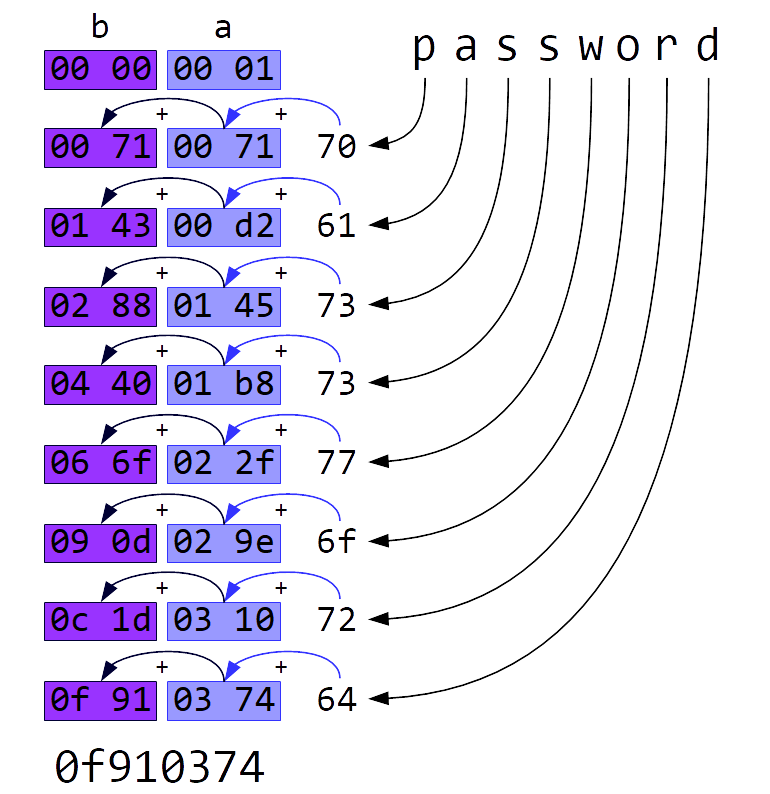Notice that `b` is initialized by `0` and `a` by `1`.

Further inspecting the code there is another function called `c`:

```  function c(a,b,c){
for(i=0;i!=a.length;i++)c=(c||'')+chr(ord(str(a[i]))^ord(str(b[i%b.length])));
return c
}
```

This functions simply encrypts/decrypts a string (`a`) using a key (`b`) by XORing every byte of the string with the corresponding byte of the key.

The outer function `x` ends with the following lines of code:

```  for(a=0;a!=1000;a++)debugger;
x=h(str(x));
source=/Ӈ#7ùª9¨M¤À.áÔ¥6¦¨¹.ÿÓÂ.Ö£JºÓ¹WþÊmãÖÚG¤¢dÈ9&òªћ#³­1᧨/;
source.toString=function(){return c(source,x)};

try{
console.log('debug',source);
with(source)return eval('eval(c(source,x))')
}catch(e){}
```

The first line runs the command `debugger` 1000 times. This command stops the execution of the current code and runs the debugger if available (e.g. `F12-tools` are open). So it does basically the same as setting a breakpoint. When debugging the code we would have to step over the command 1000 times. Of course we can easily bypass this by commenting out the line or manually setting the variable `a` to 999 in a debugger.

The second line assigns `x` the value of `h(str(x))`. Well, this is simply the adler32-checksum of the entered password. Isn’t it?

On the next line a regexp called `source` is defined, which obviously contains encrypted data. The `toString` handler of the regexp is set to `function(){return c(source,x)}`. This means that whenever the regexp-object `source` is converted to a string, this function is called.

At last, within a try/catch-block, `console.log` is called to print `source` and `eval('eval(c(source,x))')` is returned. The `return` statement is proceeded by `with(source)`, which adds `source` to the scope chain when evaluating the `return` statement.

We’ll get to the tricks behind all this in the next section.

### 3. Obfuscation / Anti-Debugging

While reading the source code for the first time, we have probably already seen some unusual statements. In this section I want to figure out, which obfuscation and anti-debugging techniques have been applied and how these can be tackled.

##### 3.1. debugger

Well, this is the most obvious anti-debugging technique. The command `debugger` is called 1000 times:

```  for(a=0;a!=1000;a++)debugger;
```

As already stated, we can comment out the line or set the value of `a` to 999 manually in a debugger.

Even though this line does not seem to be very tricky, it is not without other side-effects as we will see later!

##### 3.2. source.toString / console.log

The `toString` handler of `source` is defined to call `c(source,x)`:

```  source.toString=function(){return c(source,x)};
```

This in combination with the following line …

```    console.log('debug',source);
```

… produces an infinite loop when a debugger/console is present.

Why is that? Consider the following code:

```source=/Ӈ#7ùª9¨M¤À.áÔ¥6¦¨¹.ÿÓÂ.Ö£JºÓ¹WþÊmãÖÚG¤¢dÈ9&òªћ#³­1᧨/;
console.log(typeof(source));      // object
console.log(source.constructor);  // ƒ RegExp() { [native code] }
```

`source` is an object of type `RegExp`. When passing this object to `console.log` the regexp enclosed in slashes (`/`) is printed:

```console.log(source); // /Ӈ#7ùª9¨M¤À.áÔ¥6¦¨¹.ÿÓÂ.Ö£JºÓ¹WþÊmãÖÚG¤¢dÈ9&òªћ#³­1᧨/
```

When setting an own `toString` handler, this handler is called when the object is passed to `console.log`:

```source.toString = function() { return 'xxx'; }
console.log(source);  // xxx
```

But why does `return c(source,x)` in the `toString` handler produce an infinite loop?

Let’s have a look at the function `c` again:

```  function c(a,b,c){
for(i=0;i!=a.length;i++)c=(c||'')+chr(ord(str(a[i]))^ord(str(b[i%b.length])));
return c
}
```

The `for`-loop continues looping as long as `i!=a.length`. In this case `a` is our regexp object `source`. But wait.. What length does a regexp object have?

```source=/Ӈ#7ùª9¨M¤À.áÔ¥6¦¨¹.ÿÓÂ.Ö£JºÓ¹WþÊmãÖÚG¤¢dÈ9&òªћ#³­1᧨/;
console.log(source.length);  // undefined
```

`undefined`. This means the loop is never aborted.

But why doesn’t the `eval` statement also calling `c` stuck in an infinte loop? …

##### 3.3. with(source)

The `eval` statement is proceeded by `with(source)`:

```    with(source)return eval('eval(c(source,x))')
```

As can be read here: The with statement extends the scope chain for a statement..

Let’s have a look at a simple example:

```var sample = {'id': 1};

console.log(sample.id);       // 1
console.log(id);              // Uncaught ReferenceError: id is not defined

with(sample) console.log(id); //1
```

The object `sample` contains an attribute `id`, which can be accessed by `sample.id`. When using `with(sample)` we can directly access `id` without prepending `sample`.

This means that the statement above can also be read like this:

```    return eval('eval(c(source.source,x))')
```

The aroused confusion by the fact, that the object’s name is the same as the attribute’s name is probably not a coincidence 😉

The `RegExp.source` attribute returns a string containing the source text of the regexp object (see here):

```source=/Ӈ#7ùª9¨M¤À.áÔ¥6¦¨¹.ÿÓÂ.Ö£JºÓ¹WþÊmãÖÚG¤¢dÈ9&òªћ#³­1᧨/;
console.log(source.source);        // Ӈ#7ùª9¨M¤À.áÔ¥6¦¨¹.ÿÓÂ.Ö£JºÓ¹WþÊmãÖÚG¤¢dÈ9&òªћ#³­1᧨
console.log(source.source.length); // 55
```

This way a string is being passed to `c` and thus the `for`-loop iterates over the string’s length appropriately.

##### 3.4. encoding: x != x

While playing around with the source code, I added the following debug-message in the function `x`:

```function x(х){
console.log(x);
...
```

I assumed that the passed argument (the password being entered) would be printed, but…

```ƒ x(х){console.log(x);ord=Function.prototype.call.bind(''.charCodeAt);chr=String.fromCharCode;str=String;function h(s){for(i=0;i!=s.length;i++){a=((typeof a=='undefined'?1:a)+ord(str(s[i])))%65521;b=((…
```

… the function `x` is printed!? That should not be the case, since identifiers of passed arguments (local scope) should overwrite global scope identifiers (function `x`).

Printing a function `foo`:

```function foo(a) { console.log(foo); }
foo('qwerty');  // here foo is the function foo (global scope):
// ƒ foo(a) { console.log(foo); }
```

Passed argument with the same name overwrites global scope:

```function foo(foo) { console.log(foo); }
foo('qwerty');  // here foo is the passed argument (local scope):
// qwerty
```

After verifying this assumption, I came to the conclusion that something cannot be right with the `x` being passed and viewed the file with `hexdump`:

```user@host:~/4dead099e841668a8d86e36fcde8099ce134c195da9863dfb9039043d366942d\$ hexdump -C js_safe_2.html
00000000  3c 21 44 4f 43 54 59 50  45 20 68 74 6d 6c 3e 0a  |<!DOCTYPE html>.|
00000010  3c 68 74 6d 6c 3e 0a 3c  68 65 61 64 3e 0a 3c 6d  |<html>.<head>.<m|
00000020  65 74 61 20 63 68 61 72  73 65 74 3d 22 75 74 66  |eta charset="utf|
00000030  2d 38 22 3e 0a 3c 74 69  74 6c 65 3e 4a 53 20 73  |-8">.<title>JS s|
...
00000840  65 3e 0a 3c 73 63 72 69  70 74 3e 0a 0a 66 75 6e  |e>.<script>..fun|
00000850  63 74 69 6f 6e 20 78 28  d1 85 29 7b 6f 72 64 3d  |ction x(..){ord=|   <----
00000860  46 75 6e 63 74 69 6f 6e  2e 70 72 6f 74 6f 74 79  |Function.prototy|
...
```

The argument identifier `d1 85` is the UTF-8 representation of the cyrillic small letter ha, which looks like an `x` (`78`).

Also notice that `charCodeAt` returns `0x445`, not `0xd185` (see here):

```var cyrillic_x = 'х';
console.log(cyrillic_x.charCodeAt(0).toString(16)); // 445
```

The `x` which is being used with the checksum-function `h` is actually an `x` (`78`) and thus identifies the `function x`:

```  x=h(str(x));
```
```...
000009e0  2b 2b 29 64 65 62 75 67  67 65 72 3b 78 3d 68 28  |++)debugger;x=h(|
000009f0  73 74 72 28 78 29 29 3b  73 6f 75 72 63 65 3d 2f  |str(x));source=/|
...
```

This means, that the key to decrypt the string stored in `source` is not the checksum of the entered password, but the checksum of the stringified function `x`!

So it should actually be easy to decrypt the string:
–> calculate the checksum of the stringified function `x`
–> call the decrypt function `c` with `source.source` and the calculated checksum

```// original function x

function x(х){ord=Function.prototype.call.bind(''.charCodeAt);chr=String.fromCharCode;str=String;function h(s){for(i=0;i!=s.length;i++){a=((typeof a=='undefined'?1:a)+ord(str(s[i])))%65521;b=((typeof b=='undefined'?0:b)+a)%65521}return chr(b>>8)+chr(b&0xFF)+chr(a>>8)+chr(a&0xFF)}function c(a,b,c){for(i=0;i!=a.length;i++)c=(c||'')+chr(ord(str(a[i]))^ord(str(b[i%b.length])));return c}for(a=0;a!=1000;a++)debugger;x=h(str(x));source=/Ӈ#7ùª9¨M¤À.áÔ¥6¦¨¹.ÿÓÂ.Ö£JºÓ¹WþÊmãÖÚG¤¢dÈ9&òªћ#³­1᧨/;source.toString=function(){return c(source,x)};try{console.log('debug',source);with(source)return eval('eval(c(source,x))')}catch(e){}}

// extracted helper-functions (ord, char, str), h and c

ord=Function.prototype.call.bind(''.charCodeAt);chr=String.fromCharCode;str=String;

function h(s){for(i=0;i!=s.length;i++){a=((typeof a=='undefined'?1:a)+ord(str(s[i])))%65521;b=((typeof b=='undefined'?0:b)+a)%65521}return chr(b>>8)+chr(b&0xFF)+chr(a>>8)+chr(a&0xFF)}

function c(a,b,c){for(i=0;i!=a.length;i++)c=(c||'')+chr(ord(str(a[i]))^ord(str(b[i%b.length])));return c}

// calculate checksum of x and decrypt source string (source.source)

var source = /Ӈ#7ùª9¨M¤À.áÔ¥6¦¨¹.ÿÓÂ.Ö£JºÓ¹WþÊmãÖÚG¤¢dÈ9&òªћ#³­1᧨/;
var checksum = h(str(x));

console.log(c(source.source, checksum));
```

And the output is ..

```Ѳþ6°ä©BÁgT	¤u¸gJÃgcT¢¸K\$VÛX£-}ä'»҆"úì᧩
```

? That does not seem right. The decrypted string should be passed to `eval` once again, but this does not look like valid javascript-code. This probably means, that the checksum is not correct. So I decided to use a debugger to see how the original function `h` calculates the checksum.

##### 3.5. variable initialization: a

In order to debug the function `h`, we can simply enter a password, which matches the regexp format (e.g. `CTF{test}`) and wait for the `debugger` instruction to be reached: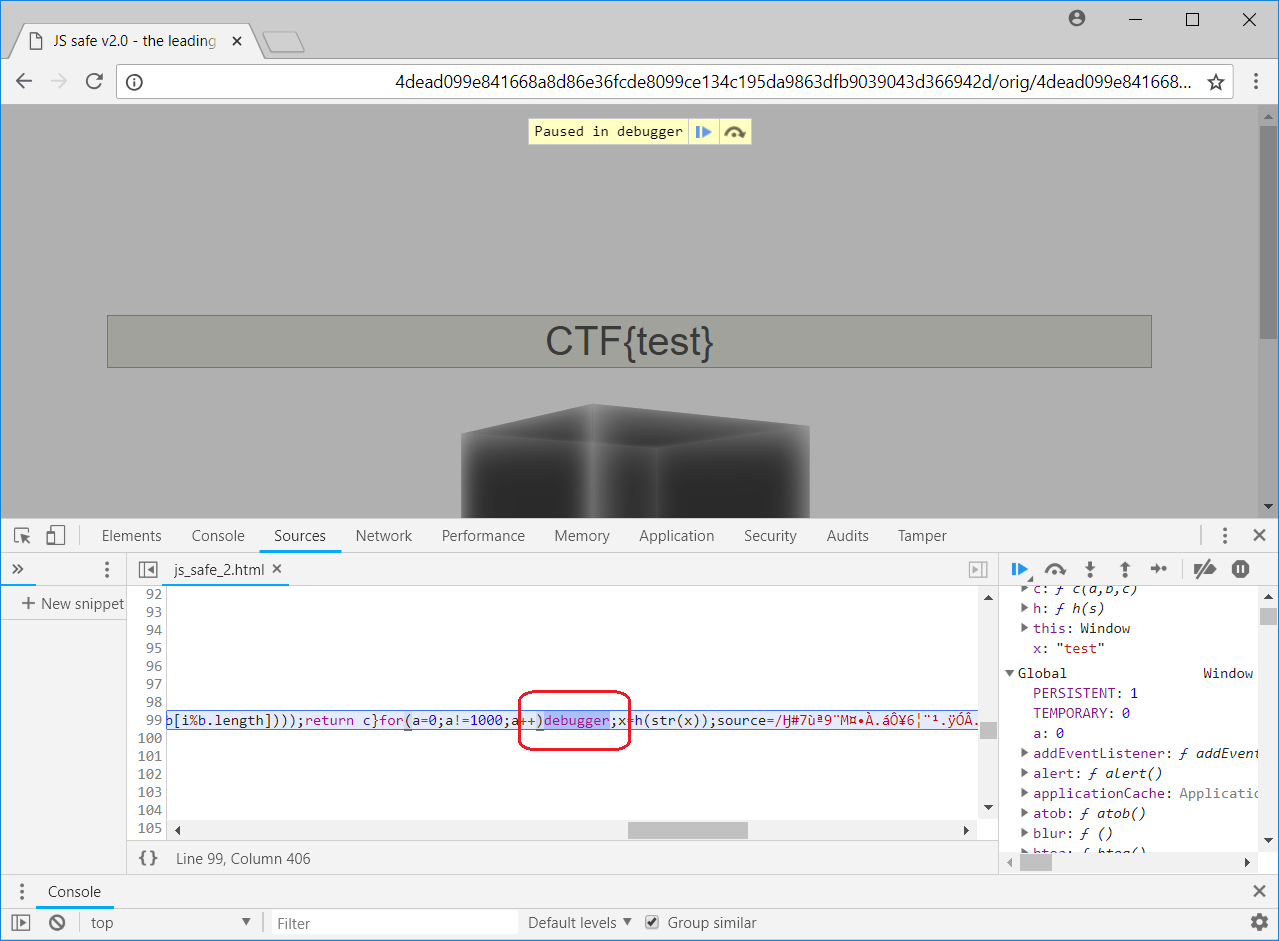Since we are stuck in the for-loop now, we can adjust the variable `a` to `999` so that the loop terminates on the next iteration: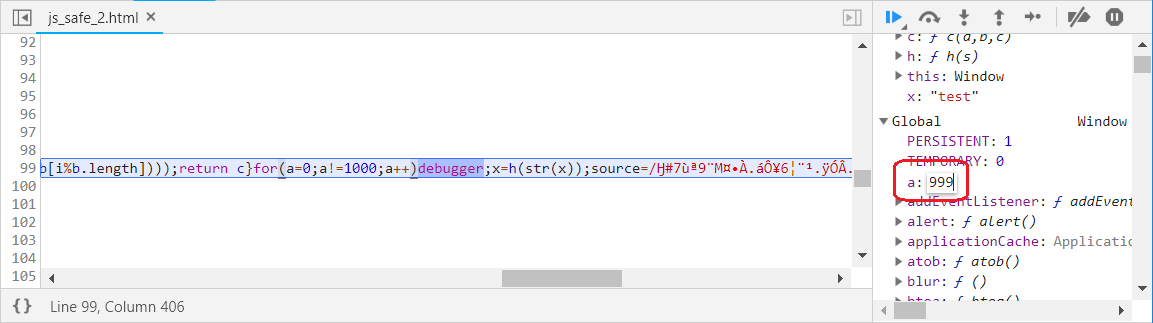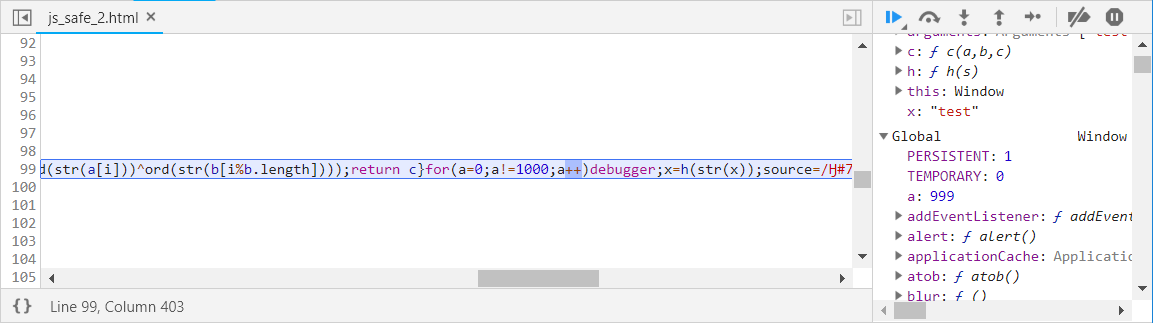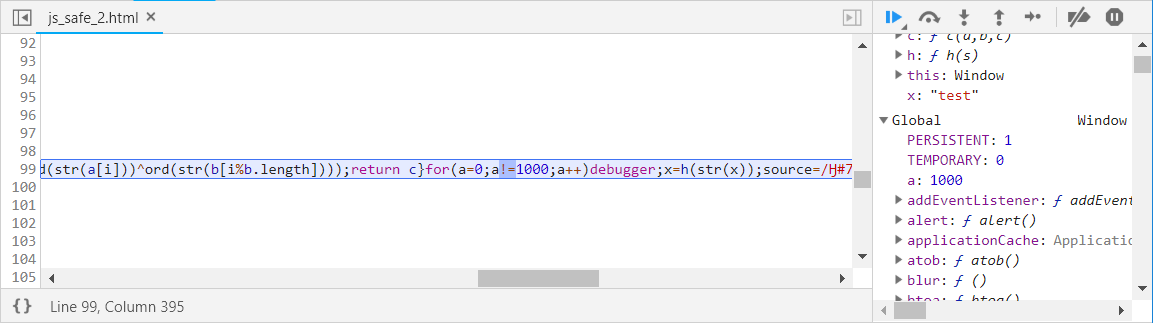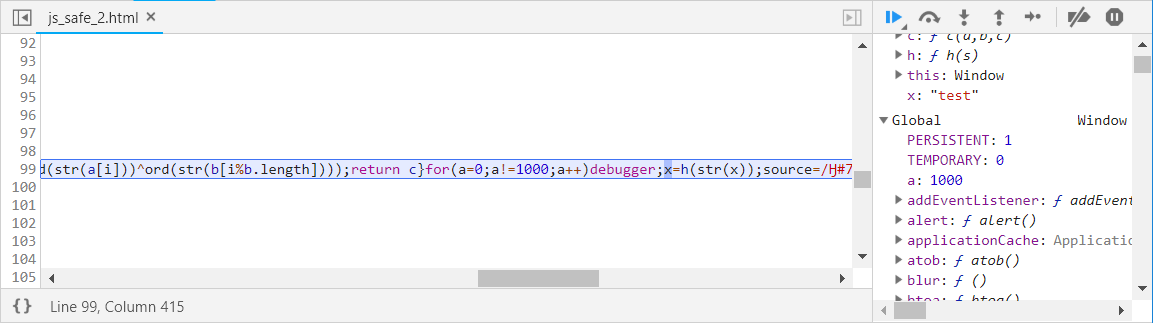We then single-step into the function `h`: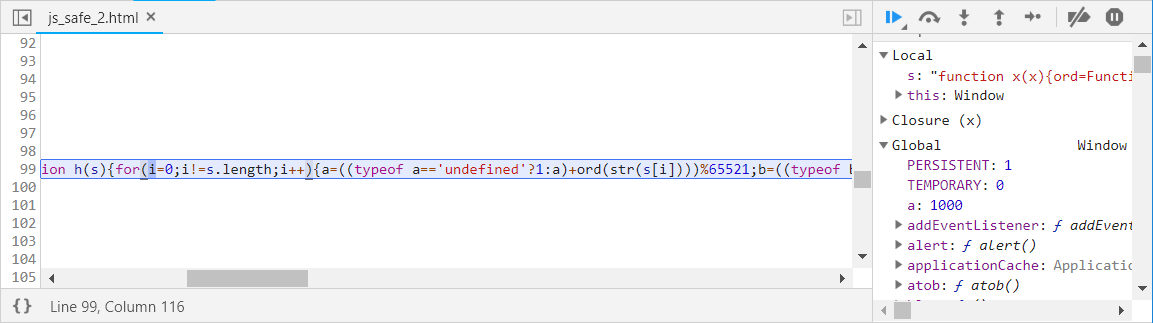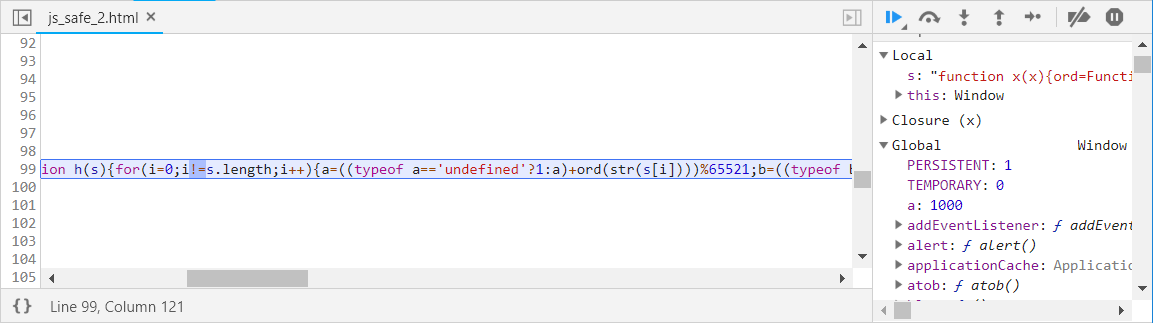Here `a` is incremented the first time: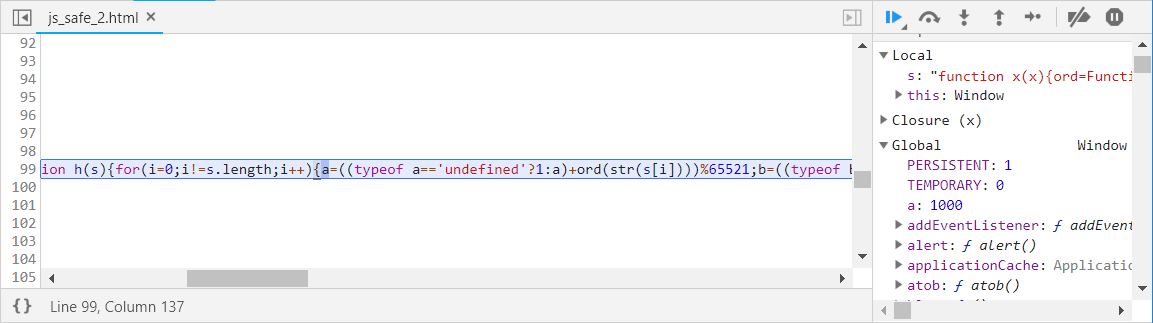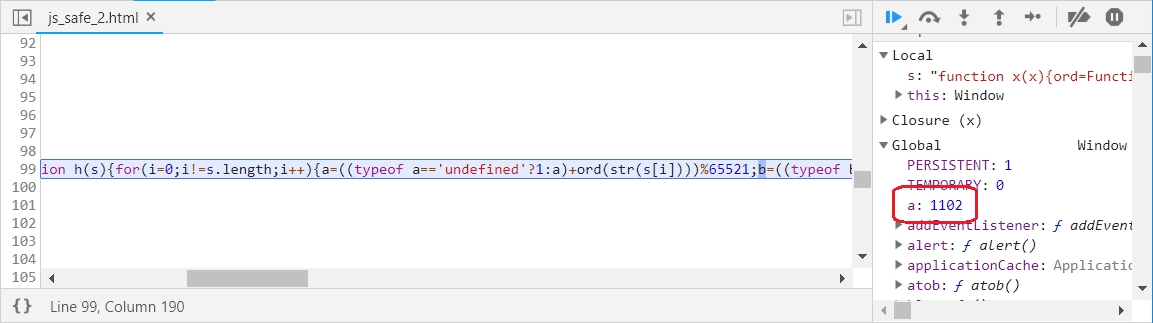What’s this? `a` is `1102` now!? Of course! `a` was already defined. Thus it hasn’t been initialized by `1`. `a` was defined within the `debugger`-loop and has the value `1000` when the function `h` is called. After the first ascii-value (`f = 102`) has been added, it’s value is `1102`.

Thus we only need to initialize `a` to `1000` in the above code:

```...
// calculate checksum of x and decrypt source

var source = /Ӈ#7ùª9¨M¤À.áÔ¥6¦¨¹.ÿÓÂ.Ö£JºÓ¹WþÊmãÖÚG¤¢dÈ9&òªћ#³­1᧨/;
var a = 1000;
var checksum = h(str(x));

console.log(c(source.source, checksum));
```

Which outputs:

```х==c('¢×&Ê´cÊ¯¬\$¶³´}ÍÈ´T©Ð8Í³Í|Ô÷aÈÐÝ&¨þJ',h(х))//᧢
```

This actually looks like valid javascript-code!

### 4. Solution

After tackling all obfuscation and anti-debugging techniques we have successfully decrypted the `source` string.

Notice that the `x` used in the decrypted javascript-code is the cyrillic x, which is the password the user entered:

```var decrypted = "х==c('¢×&Ê´cÊ¯¬\$¶³´}ÍÈ´T©Ð8Í³Í|Ô÷aÈÐÝ&¨þJ',h(х))//᧢";

function hexdump(txt) {
r = ''
for (var i = 0; i < txt.length; i++) r += txt.charCodeAt(i).toString(16) + ' ';
return r;
}

console.log(hexdump(decrypted));

// 445 3d 3d 63 28 27 a2 d7 26 81 ca b4 63 ca af ac    <-- 445 = cyrillic x
//  24 b6 b3 b4 7d cd c8 b4 54 97 a9 d0 38 cd b3 cd
//  7c d4 9c f7 61 c8 d0 dd 26 9b a8 fe 4a 27 2c 68
//  28 445 29 29 2f 2f 19e2                            <-- 445 = cyrillic x
```

Remember: our goal is to make the function `x` to return true. The returned value is `eval("х==c('¢×&Ê´cÊ¯¬\$¶³´}ÍÈ´T©Ð8Í³Í|Ô÷aÈÐÝ&¨þJ',h(х))//᧢")`. This means that `'¢×&Ê´cÊ¯¬\$¶³´}ÍÈ´T©Ð8Í³Í|Ô÷aÈÐÝ&¨þJ'` is the encrypted password we are looking for. It is encrypted using the checksum of the password itself: `h(х)`.

How can we decrypt the password?
–> We don’t know the checksum being used to encrypt the password as we simply don’t know the password.
–> We know that the key-length being used is 4.
–> We know that the decrypted password must match the regular expression and can thus only contain the following characters: `0123456789abcdefghijklmnopqrstuvwxyzABCDEFGHIJKLMNOPQRSTUVWXYZ_@!?-`

The 4-byte key is XORed with the ciphertext: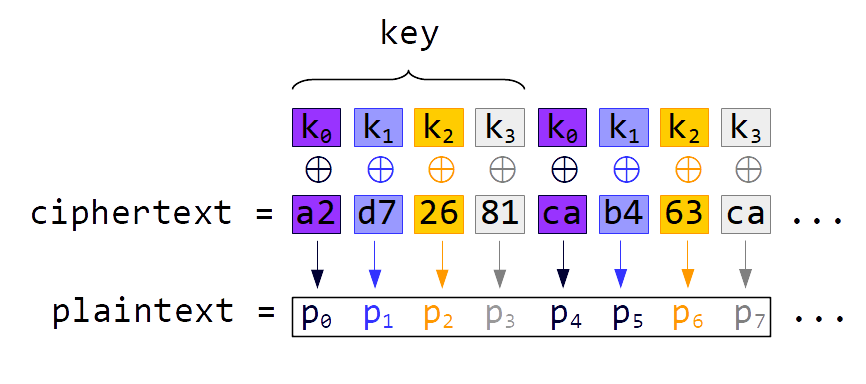Considering the mentioned assumptions we can look for possible values for each byte of the key, which produces a valid plaintext only containing characters of the allowed charset. The following picture illustrates this method:Finally I wrote the following python-script, which determines valid values for each byte of the key and tries to decrypt the encrypted password with all potentially valid key-combinations:

```user@host:~\$ cat decrypt.py
#!/usr/bin/env python

f = open('encrypted.bin')
chars = [[], [], [], []]
charset = "0123456789abcdefghijklmnopqrstuvwxyzABCDEFGHIJKLMNOPQRSTUVWXYZ_@!?-"

"""
Determines key-bytes, which produce a decrypted text containing only valid characters.
"""
def validKeys(arr):
r = []
for i in range(256):
valid = True
for a in arr:
if (chr(ord(a)^i) not in charset):
valid = False
break
if (valid): r.append(i)
return r

"""
Decrypts text by XORing with key.
"""
def decrypt(txt, k):
r = ''
for i in range(len(txt)):
r += chr(ord(txt[i])^ord(k[i%4]))
return r

# each array contains the bytes, which are XORed with the same byte of the key
for i in range(len(encr)):
chars[i%4].append(encr[i])

# find best solution for each key-byte
keys = []
for c in chars:
keys.append(validKeys(c))

# print possible solutions using the calculated keys
for k0 in keys:
for k1 in keys:
for k2 in keys:
for k3 in keys:
key = chr(k0)+chr(k1)+chr(k2)+chr(k3)
print("[ key = "+key.encode("hex")+ " ]:"),
print(decrypt(encr, key))

```

Running the script yields two possible passwords:

```user@host:~\$ ./decrypt.py
[ key = fd9515f9 ]: _B3x7!v3R91ON!h45!AnTE-4NXi-abt1-H3bUk_
[ key = fd9915f9 ]: _N3x7-v3R51ON-h45-AnTI-4NTi-ant1-D3bUg_
```

Obviously the second one makes sense. Thus the flag is `CTF{_N3x7-v3R51ON-h45-AnTI-4NTi-ant1-D3bUg_}`.

The following picture illustrates the relation of all involved strings / checksums.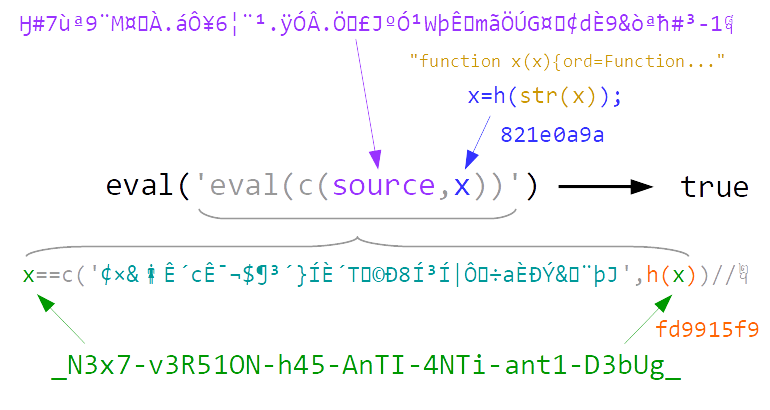Done! A really great challenge 🙂